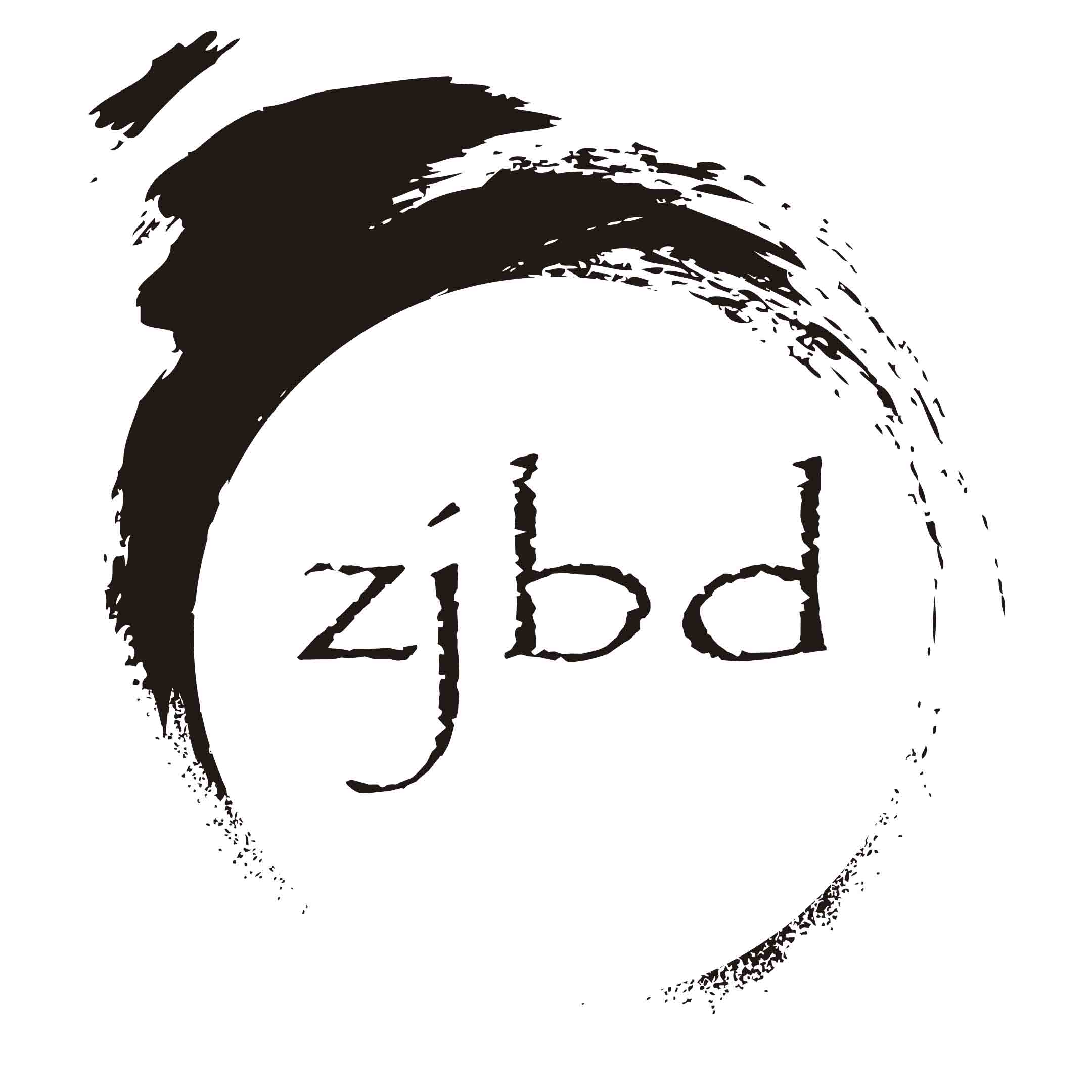PHP类型检测

## 1.is_bool(\$a)

``````检测\$a是否为true或false(布尔型)
``````

## 2.is_integer(\$a)

``````检测\$a是否为整型
``````

## 3.is_double(\$a)

``````检测\$a是否为浮点数(双精度型)
``````

## 4.is_float(\$a)

``````检测\$a是否为浮点型
``````

## 5.is_string(\$a)

``````检测\$a是否为字符数据(字符串类型)
``````

## 6.is_object(\$a)

``````检测\$a是否为对象类型
``````

## 7.is_array(\$a)

``````检测\$a是否为数组类型
``````

## 8.is_resource(\$a)

``````检测\$a是否为用于识别和处理外部资源(如数据库或文件)的句柄(资源类型)
``````

## 7.is_null(\$a)

``````检测\$a是否为未分配的值(NULL空类型)
``````

## 8.is_scalar(\$a)

``````检测\$a是否是标量类型(int、float、string、boolean)
``````

## 9.is_numeric(\$a)

``````检测\$a是否位数字或数字字符串
``````

## 10.is_callable(\$a)

``````检测\$a是否能够进行函数调用(如:回调函数),需要注意的是is_callable()函数可以传数组，第一个元素表示对象，第二个元素表示方法名，假如存在也可以通过检测。
``````

## 外送两个函数

``````gettype(\$a); 获取\$a的类型
settype(\$a, 'bool') 设置变量的类型(类似强制转换)``````

点赞 4
收藏 0•123123
回复

2015年
7月